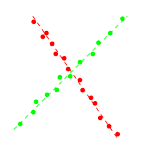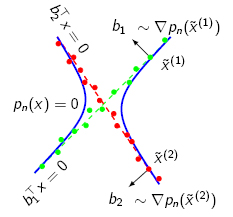GENERALIZED PCA
Project summary

Principal Component Analysis (PCA) refers to the problem of fitting a linear subspace S in RD of unknown dimension d < D to N sample points {xj}, j=1,...,N drawn from S. This problem shows up in a variety of applications in many fields, e.g., pattern recognition, data compression, regression, image processing, etc., and can be solved in a remarkably simple way from the singular value decomposition (SVD) of the (mean-subtracted) data matrix [x1, x2, ..., xN] in RD x N.

Generalized Principal Component Analysis is an extension of PCA where the data X={xj}, j=1,...,N, is assumed to be drawn from a collection of n >= 1 different linear subspaces Si in RD, i=1,...,n of dimension di = dim(Si), 0 < di < D. An example for n=2 and D=2 is shown in the figure below.The task is to find the number of subspaces, their dimensions, a basis for each subspace, and the segmentation of the data, without knowing which data point belong to which subspace.

Most existing subspace clustering algorithms of the iterative algorithms try to find a solution by iterating between two steps: given an estimated clustering of the points, find best subspace for each group; given the subspaces, cluster the data accordingly. Unfortunately, this iterative procedure often converges to a local minimum, so the initialization is critical in order to find a good solution.

GPCA is an algebraic technique that avoids this problem and bypasses the iteration steps by fitting a single model (a polynomial) which represents the union of all subspaces (i.e., the model will be satisfied by a point belonging to anyone of the subspaces). This global model is then factorized into the single models to obtain a basis for each one of the subspaces.

PROBLEM DESCRIPTION

GPCA is an algebraic subspace clustering technique based on polynomial fitting and differentiation. The key idea is to represent the number of subspaces as the degree of a polynomial and encode the subspace bases as factors of these polynomials.

Basic GPCA Algorithm

For the sake of simplicity, assume that the data was distributed on a collection of two hyperplanes, each of one can be represented by the equation xTbi=0. By multiplying the two equations for the two subspaces, we obtain

(xTb1)(xTb2)=p(x)=0
where p(x) is a homogeneous polynomial of degree two in the coordinates of x which is satisfied by all data points, regardless of which hyperplane they belong to. Therefore this polynomial can be considered as a global model representing all subspaces.

Fitting of the polynomial

As with any polynomial, p(x) may be expanded linearly in terms of some coefficients with respect to a basis of polynomials. Therefore, given enough data points from the subspaces, the coefficients of p(x) can be linearly estimated from the data by using a non-linear embedding (the Veronese map). Building upon our previous example, assume that x lives in R3, say x=[x,y,z]T. Then, p(x) can be expanded as

p(x)=ax2+by2+cz2+dxy+eyz+fxz.
It is immediate to note that the polynomial is non-linear in the coordinates x,y,z but is linear in the coefficients a,b,c,d,e,f. The latter can be therefore retrived (up to a scale factor) by solving the homogeneous linear system
p(xi)=0,   i=1,...,n.

Basis extraction by differentiation

Now, the set of points x such that p(x)=0 can be thought of as a surface (in our previous example, p(x) is the equation of a conic in the projective plane P2). Moreover, the gradient of p(x), ∇p(x) must be orthogonal to this surface. Since the surface is a union of subspaces, the gradient at a point must be orthogonal to the subspace passing through that point. As a result, we may compute the normal vectors at a point as the gradient of p(x) at that point, as illustrated in the figure below.Since all points in the same cluster should have the same (or similar) normal vectors, we can cluster the data by clustering these normal vectors. In fact, the angles between these normal vectors provide use with a similarity measure that can be used together with spectral clustering.

Extensions of GPCA
Clustering subspaces of different dimensions

In the case of hyperplanes, a single polynomial is enough, but in the case of subspaces of different dimensions, it is necessary to fit more polynomials. The gradient of each one of these polynomials at a point x will give possibly a different normal vector. All these normal vectors will form a basis for the complement of the subspace passing through x. The principal angles between these orthogonal subspaces may now be used to define a similarity measure, and clustering proceeds as before. More details can be found in the references.

Dealing with noise and outliers

GPCA is an algebraic technique which provide the exact algebraic solution in closed form when the data is perfect. With a moderate amount of noise, GPCA will still operate correctly, as the polynomials can be estimated using least squares. However, in the presence of large amounts of noise and outliers, the coefficients may be estimated incorrectly. For this reason, some variations of the main algorithm have been developed. Some of these employ spectral clustering, voting schemes, multivariate-trimming and influence functions to deal with non-perfect data. Please refer to the page from UIUC for more details and code samples on this topic.

Code

An implementation of GPCA in Matlab (with applications to motion segmentation) is also available from our group. See the Code page.

Applications of GPCA and publications
GPCA has been successfully applied in various field of computer vision. In the following we present a (non-exaustive) list of applications that are based on the ideas of GPCA.
Subspace clustering

IEEE Conference on Computer Vision and Pattern Recognition, 2003.

IEEE Conference on Computer Vision and Pattern Recognition, 2004.

IEEE Conference on Computer Vision and Pattern Recognition, 2004

IEEE Transactions on Pattern Analysis and Machine Intelligence, 2005.

International Conference on Machine Learning, 2006

A ZGPCA Algorithm for Subspace Estimation
IEEE International Conference on Multimedia and Expo, 2007

Contextual Distance for Data Perception
IEEE International Conference on Computer Vision, 2007

Estimation of Subspace Arrangements with Applications in Modeling and Segmenting Mixed Data,
Preprint, to appear in SIAM Review, 2007
Hilbert series of subspace arrangements

Hilbert Functions and Applications to the Estimation of Subspace Arrangements
IEEE International Conference on Computer Vision, 2005

Hilbert series of subspace arrangements
Journal of Pure and Applied Algebra, 2007
Motion segmentation

IEEE Workshop on Vision and Motion Computing, 2002

IEEE Conference on Computer Vision and Pattern Recognition, 2003

IEEE Conference on Computer Vision and Pattern Recognition, 2004

Motion Segmentation of Multiple Translating Objects Using Line Correspondences
IEEE Computer Society Conference on Computer Vision and Pattern Recognition, 2005

A Factorization-Based Approach for Articulated Non-rigid Shape, Motion and Kinematic Chain Recovery
IEEE Conference on Computer Vision and Pattern Recognition, 2005

Journal of Mathematical Imaging and Vision, 2006

International Journal of Computer Vision, 2006

Imaging Beyond the Pinhole Camera, Springer, 2006

IEEE International Conference on Computer Vision and Pattern Recognition, 2007

IEEE Transactions on Pattern Analysis and Machine Intelligence, 2007

3D Motion Segmentation from Straight-Line Optical Flow
Multimedia Content Analysis and Mining, Springer, 2007
Hybrid Systems

IEEE Conference on Decision and Control, 2003

IEEE Conference on Decision and Control, 2004

IEEE Conference on Decision and Control, 2005

European Control Conference, 2007

Recursive Identification of Switched ARX Systems
Automatica, 2007
Dynamic Textures

IEEE Conference on Computer Vision and Pattern Recognition, 2005

Dynamical Vision Workshop in the European Conf. on Computer Vision, 2006

Spatial segmentation of temporal texture using mixture linear models
Dynamical Vision, Springer, 2007
Image segmentation and compression

GPCA: an efficient dimension reduction scheme for image compression and retrieval
ACM SIGKDD international conference on Knowledge discovery and data mining, 2004

A Multi-Scale Hybrid Linear Model for Lossy Image Representation
IEEE International Conference on Computer Vision, 2005

Segmenting Small Regions in the Presence of Noise
IEEE International Conference on Image Processing, 2005

Scale adaptive visual attention detection by subspace analysis
International Conference on Multimedia, 2007
Biomedical imaging

Segmenting a Beating Heart Using Polysegment and Spatial GPCA
IEEE International Symposium on Biomedical Imaging: Nano to Macro, 2006
Vision-Based Formation Control

Following the Flock: Distributed Formation Control with Omnidirectional Vision-Based Motion Segmentation and Visual Servoing.
IEEE Robotics and Automation Magazine, 2004
Please refer to the specific webpages on this same website for more details on this topic.